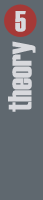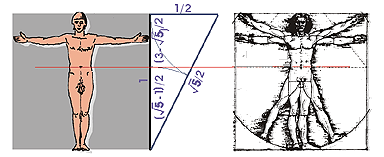Theory is important for understanding

Theory points out uniformity of construction and variety of external forms. The sections are unavoidably triangular, running from the middle of the wall till the ridge. The baseline equals the internal diameter of the space plus two halves of the walls thickness and is measured from the entrance as the walls' thickness plus the internal depth of useful space. The height of the triangle is the root of three halves, but can also be composed from three equally long sticks used as toys by shepherds during pasture. Thus theory coupled with mathematics joins with practice of the simple builders' development that uses theoretical principles although not even being aware of their existence.

Corbelling is a construction more than six thousand years old: the Hypogeum in Malta shows it in the carved subterranean sanctuary built more than four thousand years ago.Man and proportions: man's proportion is golden section, and is in use at the stone shelters, but for shelter is equilateral triangle typical

Hereby the most important element affecting composition and its recognisable order is the relation of dimensions. Relations vary, from simple to highly complex ones, but the most effective ones are those, which can be easily constructed; these are the most distinct and easily remembered.

From the circle and derived square (internal or external) the relation with square root of two emerges. The square root of two is the diagonal of a square whose baseline is one. Paper used in day-to-day practice is such.

The gold section is a limitless relation, both in enlargement and reduction. It is built on the human scale, thus is easily acceptable. It is however difficult to express, by using two squares and the diagonal of two squares, which equals the square root of five. The gold section is seen in nature, both in detail and in whole entities, even in space.
The sides of an Egyptian triangle measure 3, 4 and 5 and it has one right angle. In times primeval Egyptians determined right angles with it when working in the desert. Today we know it as the relations of a television screen.Theory in practice: triangle, inscribed on the top stone simple shepherds play with three sticks: triangle, left: Grans (Provence), France

The corbelling construction brings a new relation: the equilateral triangle. It's height equals the baseline multiplied by the half of the square root of three, as was proven by Pythagoras.

Shepherds played with wooden sticks: from three equally long sticks they composed the only possible form: the equilateral triangle.
By using three sticks we can also determine the half of the square root of three. This is the height of corbelling up till the ridge.

The principle isn't only an aid in construction: by opposite use we can determine the height of construction built by corbelling quite accurately. Theoretical analysis is proven even in practice.Practical work at corbelling: diameter of groundplan, baseline and height of equilateral triangle are the elements of constructing the shelter in corbelling